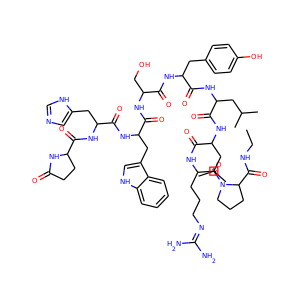877-202-0205     support@chemchart.com     @chemchartLeuprorelin
Weight 1209.421 g/mol C59H84N16O12 14 15 4 32

# Leuprorelin (53714-56-0, 74381-53-6)

"What do you need help with?"### Alternate Names

• SMILES
CCNC(=O)C1CCCN1C(=O)C(CCCN=C(N)N)NC(=O)C(CC(C)C)NC(=O)C(CC(C)C)NC(=O)C(CC2=CC=C(C=C2)O)NC(=O)C(CO)NC(=O)C(CC3=CNC4=CC=CC=C43)NC(=O)C(CC5=CN=CN5)NC(=O)C6CCC(=O)N6
• InChIKey
GFIJNRVAKGFPGQ-UHFFFAOYSA-N